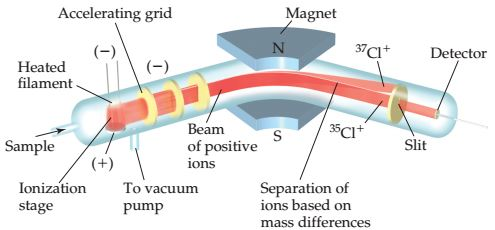# Problem: Consider the mass spectrometer show below.Determine whether each of the following statements is true or false.The height of each peak in the mass spectrum is directly proportional to the relative abundance of the isotope.

###### FREE Expert Solution

We’re being asked to determine if the following statement is true:

The height of each peak in the mass spectrum is directly proportional to the relative abundance of the isotope

Mass Spectrometry allows you to determine the molecular weight of an unknown compound through its vaporization and ionization. A mass spectrometer is used to determine the mass to charge ratio of an unknown compound.

Given: The height of each peak in the mass spectrum is directly proportional to the relative abundance of the isotope.

81% (264 ratings)###### Problem Details

Consider the mass spectrometer show below.Determine whether each of the following statements is true or false.

The height of each peak in the mass spectrum is directly proportional to the relative abundance of the isotope.